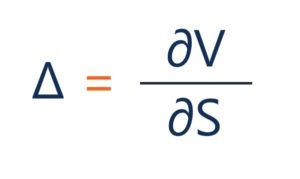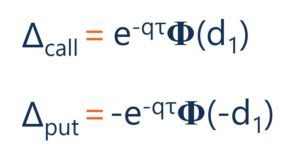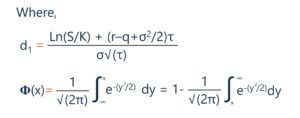# Delta (Δ)

The sensitivity of a derivative's price to the underlying asset value

## What is Delta?

Delta is a risk sensitivity measure used in assessing derivatives. It is one of the many measures that are denoted by a Greek letter. The series of risk measures that use such letters are fittingly referred to as the Greeks. They are often also called risk measures, hedge parameters, or risk sensitivities.Of the Greeks, delta is one of the most important metrics. It compares the change in the price of a derivative to the changes in the underlying asset’s price. For example, a long call option with a delta of 0.30 would rise by \$0.30 if the underlying asset rose in price by \$1. Traders often refer to the sensitivity measure in basis points. A delta of 0.30 may be referred to as “30 delta.”

### Quick Summary of Points

• Delta is a risk sensitivity measure used in assessing derivatives.
• The sensitivity measure is equal to the change in the derivative value as a ratio of the change in the underlying asset’s price.
• Delta can be used for a number of purposes, including gauging risk, exposure, and hedging.

### How to Interpret Delta?

Delta can be thought of as a ratio that compares changes in the derivative price and the underlying asset price. The ratio can be positive or negative depending on the direction the derivative moves in relation to changes in the underlying asset. A call option would increase in value if the underlying asset rose in price; therefore, it has a positive delta. A put option would decrease in value if the underlying asset rose in price; therefore, it has a negative delta. For a call option, the value will range from 0 to 1, and for a put option, the value will range from 0 to -1.

Let us look at an example of this ratio. Say a call option has a value of \$10, and the underlying asset has a price of \$20. The underlying asset increases in price to \$23, and the option value corresponds by increasing to \$11. The delta is equal to: (\$11-\$10)/(\$23-\$20) = 0.33.

Now let us look at a put option with a value of \$10. The underlying asset has a price of \$20 and decreases to \$17. The corresponding put option value increases to \$11. The delta here is equal to: (\$11-\$10)/(\$17-\$20) = -0.33.

Another way of thinking about the metric is that it can give an idea of whether an option will end up in the money at the expiration date. As an option moves further into the money, the delta value will head away from 0. For a call option, it will head toward a value of 1, while a put option will head toward a value of -1. As the option moves further out of the money, the delta value will head towards 0.

The delta is 0.50 when a call option is at the money and -0.5 for a put option when it is at the money, meaning the strike price is equal to the underlying asset’s price. It is essentially saying there is a 50/50 chance of the option ending in the money or out of the money.

The delta sensitivity is also affected by the time until expiration. The closer the option is to expiration, the more likely the option will end up in its current state whether in, out, or at the money. If a call option is in the money, holding the moneyness constant, as it approaches expiration, the delta value will move closer towards one.

### What is Delta Used For?

This sensitivity measurement is one of the most important Greeks used in assessing derivatives. As mentioned before, it can be thought of as the probability that an option will end in the money. It is very important to consider when determining the risk one is willing to take for a return of an investment. Observing the value in such a way can also be used in assessing portfolios. Looking at the portfolio aggregate delta can help determine how it would do in relation to changes in the overall market.

Traders may consider the sensitivity value as the amount of their exposure to a stock or the underlying asset. The closer to 1 the value is, the more exposed they are to the underlying asset.

The delta value of an option can also be used as a way to determine whether the options are being bought or sold. If the price of an option increases less than the delta would imply, it could mean that traders are selling this option near the bid price. If the price is higher than the delta would imply, it could mean traders were buying the options near the ask price.

Delta can also be used for hedging purposes. A common hedging strategy used is the neutral delta strategy. It involves holding a number of options that when the delta is taken in aggregate, it is equal or very close to 0. This reduces the movement in options pricing relative to the underlying asset’s price.

### How is Delta Calculated?

Delta can be shown in its general form:Where:

• ∂ – the first derivative
• V – the option’s price (theoretical value)
• S – the underlying asset’s price

Under the Black-Scholes model, delta is calculated by the following equation:Where:

• S – the stock price
• K – the strike price
• r – the risk-free rate
• q – the annual dividend yield
• τ – time until expiration
• σ – the volatility

### Additional Resources

Thank you for reading CFI’s article on delta. If you would like to learn about other related concepts, check out CFI’s other resources:

• Option Greeks
• Derivatives
• Options Case Study – Long Call
• Long and Short Positions

### Corporate Finance Training

Advance your career in investment banking, private equity, FP&A, treasury, corporate development and other areas of corporate finance.

Enroll in CFI’s Finance Courses to take your career to the next level! Learn step-by-step from professional Wall Street instructors today.